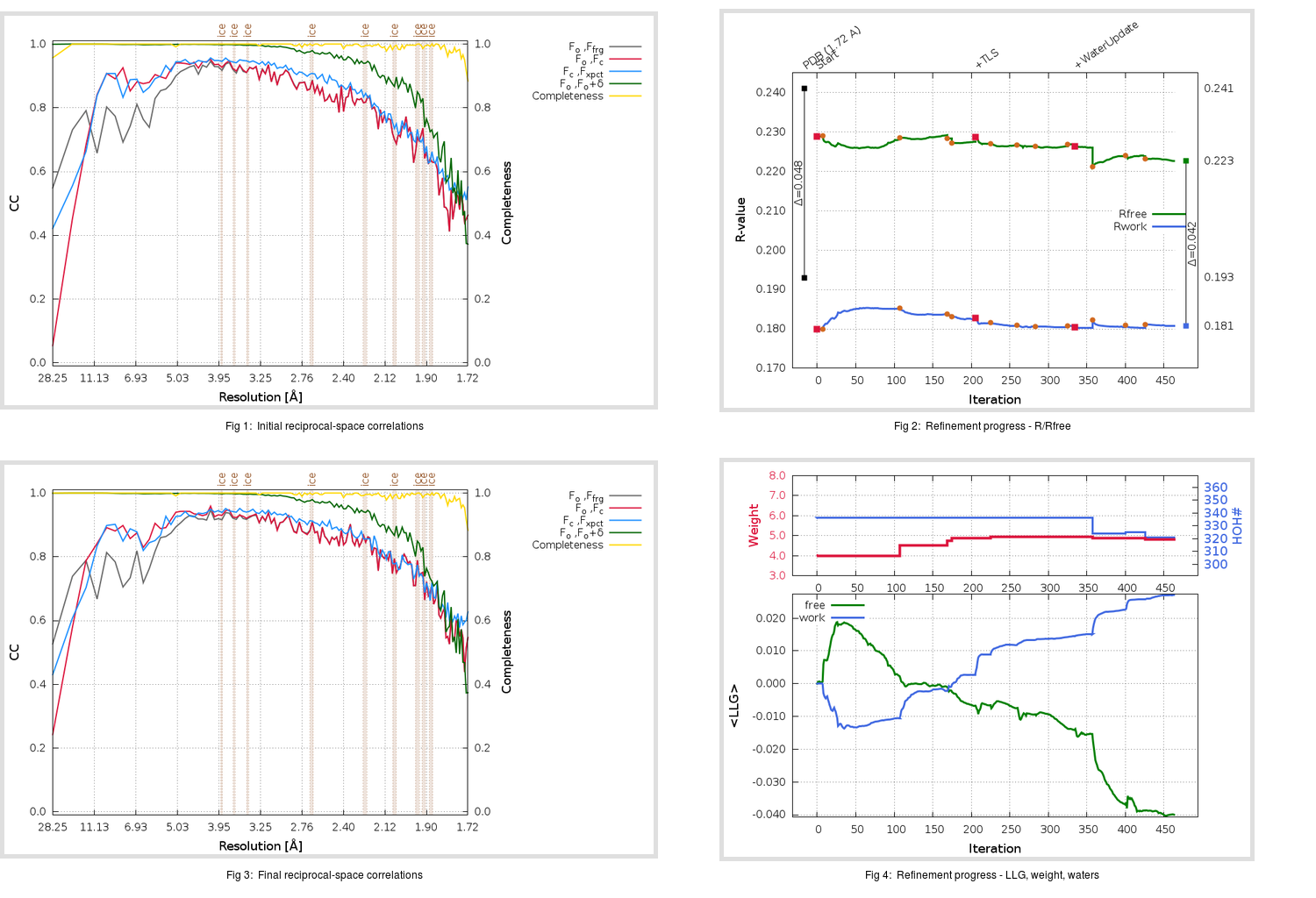Content:

```    Diffraction limits & principal axes of ellipsoid fitted to diffraction cut-off surface:
1.954         0.9119   0.0000  -0.4105       0.967 a* - 0.253 c*
1.649         0.0000   1.0000   0.0000       b*
1.710         0.4105   0.0000   0.9119       0.793 a* + 0.609 c*
```

## Deposited

` `
 Date deposited Date data collection Resolution R, Rfree 20200315 20200303 1.72 0.1900 0.2410

Molprobity (CCP4 7.0 version) summary:

```Ramachandran outliers =   0.33 %
favored =  98.01 %
Rotamer outliers      =   0.76 %
C-beta deviations     =     0
Clashscore            =   5.27
RMS(bonds)            =   0.0131
RMS(angles)           =   1.81
MolProbity score      =   1.28
Resolution            =   1.72
R-work                =   0.1900
R-free                =   0.2410
```

```Number of waters      =   336

<B> (all atoms) =   24.20 ( sd =    9.23 ) for       2739 non-hydrogen atoms
<B>   (protein) =   22.70 ( sd =    8.32 ) for       2370 non-hydrogen atoms
<B>     (water) =   33.75 ( sd =    9.47 ) for        336 non-hydrogen atoms
<B>    (others) =   36.46 ( sd =    9.98 ) for         33 non-hydrogen atoms

B min/max       (all non-hydrogen atoms) =   10.29 /   69.98
B min/max   (protein non-hydrogen atoms) =   11.61 /   69.98
B min/max     (water non-hydrogen atoms) =   10.29 /   63.12
B min/max     (other non-hydrogen atoms) =   24.57 /   51.18
```

## BUSTER (re-)refinement

` `

Molprobity (CCP4 7.0 version) summary:

```Ramachandran outliers =   0.33 %
favored =  98.68 %
Rotamer outliers      =   0.76 %
C-beta deviations     =     0
Clashscore            =   3.16
RMS(bonds)            =   0.0117
RMS(angles)           =   1.56
MolProbity score      =   1.11
Resolution            =   1.72
R-work                =   0.1808
R-free                =   0.2226
```

```Number of waters      =   321

<B> (all atoms) =   25.66 ( sd =    8.65 ) for       2724 non-hydrogen atoms
<B>   (protein) =   24.04 ( sd =    7.25 ) for       2370 non-hydrogen atoms
<B>     (water) =   36.59 ( sd =    9.82 ) for        321 non-hydrogen atoms
<B>    (others) =   36.19 ( sd =   10.48 ) for         33 non-hydrogen atoms

B min/max       (all non-hydrogen atoms) =   13.63 /   65.26
B min/max   (protein non-hydrogen atoms) =   13.63 /   65.26
B min/max     (water non-hydrogen atoms) =   14.15 /   61.82
B min/max     (other non-hydrogen atoms) =   28.52 /   43.16
```

Refinement progression:Results:

` `
 File Remark 5REJ_aB_refine.01_03_refine.pdb.gz exact refinement commands are in header 5REJ_aB_refine.01_03_refine.mtz.gz including original deposited data and several re-refinement map coefficients 5REJ_aB_refine.01_03_BUSTER_model.cif.gz including any non-standard compound restraints 5REJ_aB_refine.01_03_BUSTER_refln.cif.gz